Examples

Chapter 10 Class 12 Vector Algebra
Serial order wise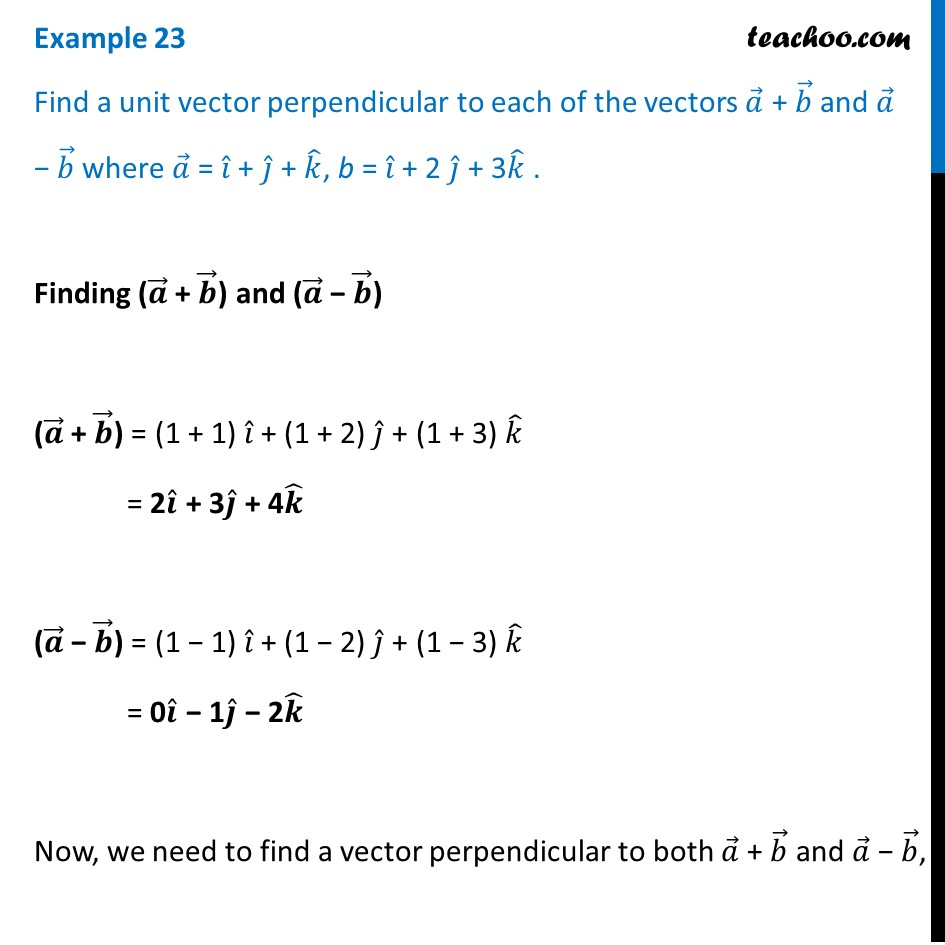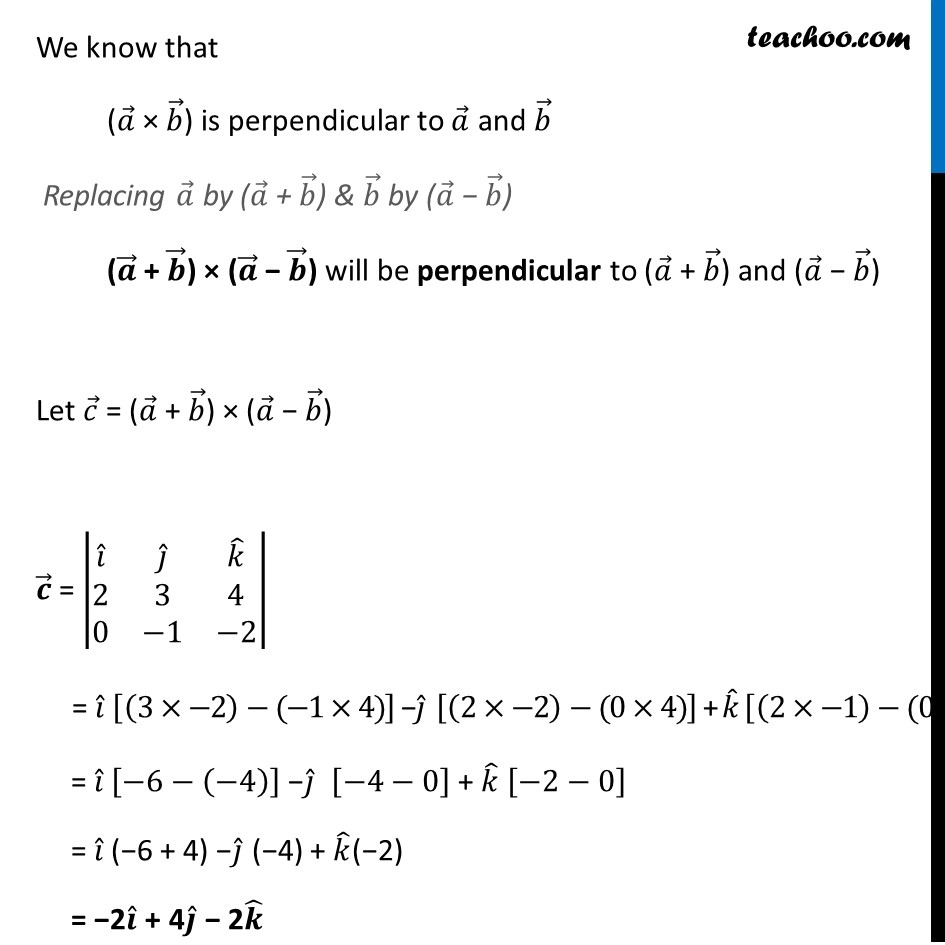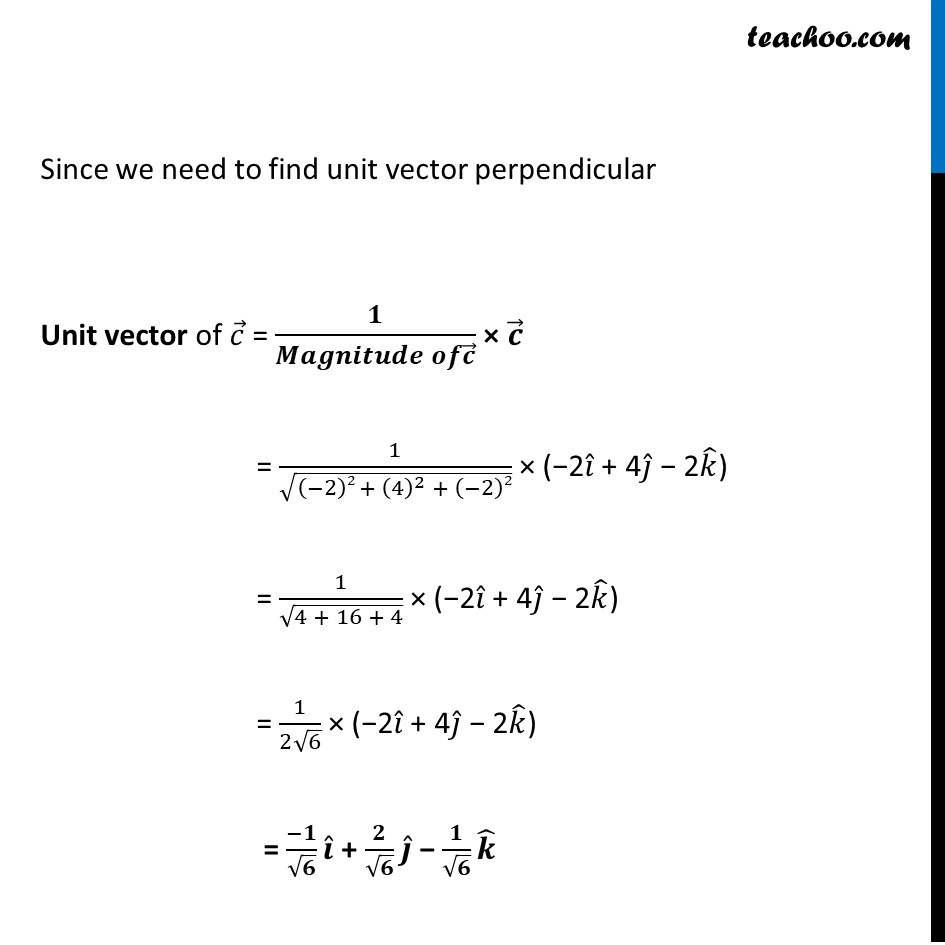This video is only available for Teachoo black users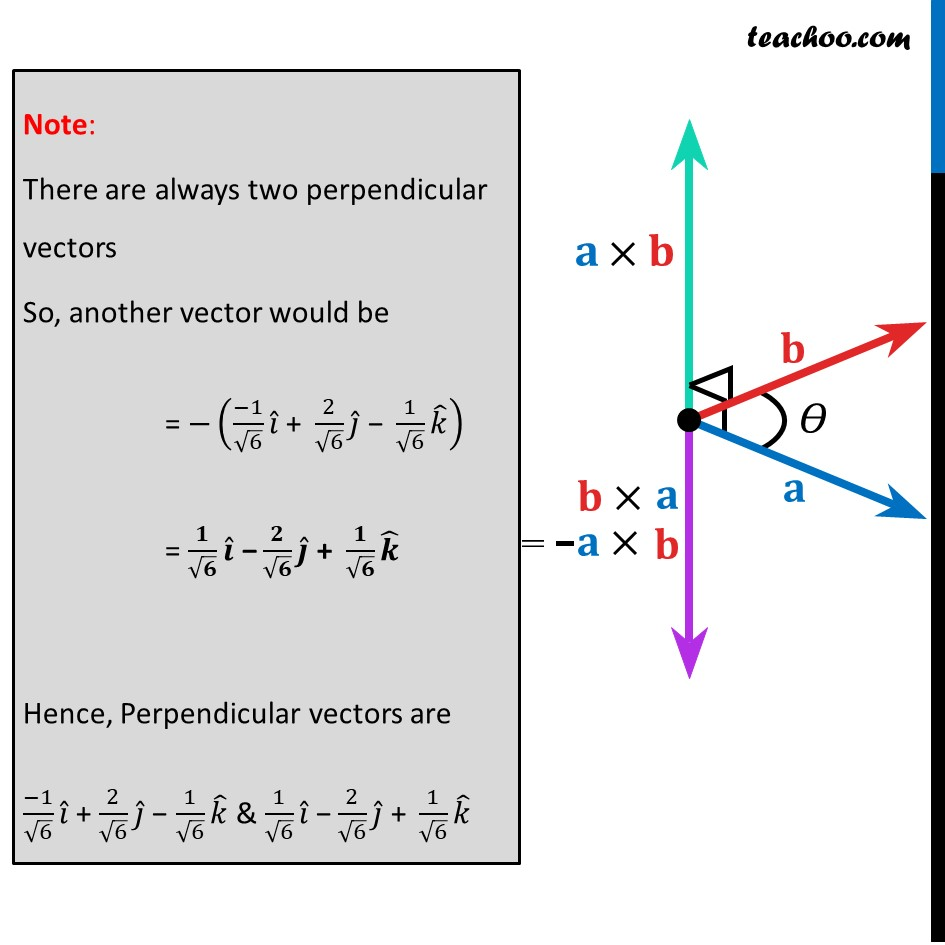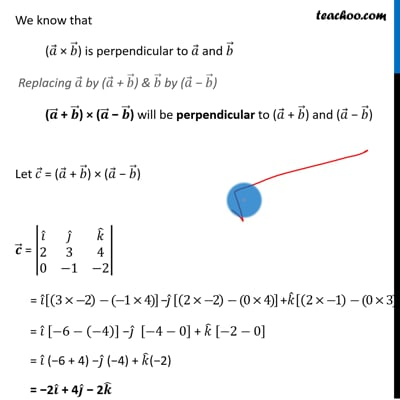This video is only available for Teachoo black users

Learn in your speed, with individual attention - Teachoo Maths 1-on-1 Class

### Transcript

Example 23 Find a unit vector perpendicular to each of the vectors 𝑎 ⃗ + 𝑏 ⃗ and 𝑎 ⃗ − 𝑏 ⃗ where 𝑎 ⃗ = 𝑖 ̂ + 𝑗 ̂ + 𝑘 ̂, b = 𝑖 ̂ + 2 𝑗 ̂ + 3𝑘 ̂ . Finding (𝒂 ⃗ + 𝒃 ⃗) and (𝒂 ⃗ − 𝒃 ⃗) (𝒂 ⃗ + 𝒃 ⃗) = (1 + 1) 𝑖 ̂ + (1 + 2) 𝑗 ̂ + (1 + 3) 𝑘 ̂ = 2𝒊 ̂ + 3𝒋 ̂ + 4𝒌 ̂ (𝒂 ⃗ − 𝒃 ⃗) = (1 − 1) 𝑖 ̂ + (1 − 2) 𝑗 ̂ + (1 − 3) 𝑘 ̂ = 0𝒊 ̂ − 1𝒋 ̂ − 2𝒌 ̂ Now, we need to find a vector perpendicular to both 𝑎 ⃗ + 𝑏 ⃗ and 𝑎 ⃗ − 𝑏 ⃗, We know that (𝑎 ⃗ × 𝑏 ⃗) is perpendicular to 𝑎 ⃗ and 𝑏 ⃗ Replacing 𝑎 ⃗ by (𝑎 ⃗ + 𝑏 ⃗) & 𝑏 ⃗ by (𝑎 ⃗ − 𝑏 ⃗) (𝒂 ⃗ + 𝒃 ⃗) × (𝒂 ⃗ − 𝒃 ⃗) will be perpendicular to (𝑎 ⃗ + 𝑏 ⃗) and (𝑎 ⃗ − 𝑏 ⃗) Let 𝑐 ⃗ = (𝑎 ⃗ + 𝑏 ⃗) × (𝑎 ⃗ − 𝑏 ⃗) 𝒄 ⃗ = |■8(𝑖 ̂&𝑗 ̂&𝑘 ̂@2&3&4@0&−1&−2)| = 𝑖 ̂ [(3×−2)−(−1×4)] −𝑗 ̂ [(2×−2)−(0×4)] + 𝑘 ̂ [(2×−1)−(0×3)] = 𝑖 ̂ [−6−(−4)] −𝑗 ̂ [−4−0] + 𝑘 ̂ [−2−0] = 𝑖 ̂ (−6 + 4) −𝑗 ̂ (−4) + 𝑘 ̂(−2) = −2𝒊 ̂ + 4𝒋 ̂ − 2𝒌 ̂ Since we need to find unit vector perpendicular Unit vector of 𝑐 ⃗ = 𝟏/(𝑴𝒂𝒈𝒏𝒊𝒕𝒖𝒅𝒆 𝒐𝒇𝒄 ⃗ ) × 𝒄 ⃗ = 1/√((−2)2 + (4)^2 + (−2)2) × (−2𝑖 ̂ + 4𝑗 ̂ − 2𝑘 ̂) = 1/√(4 + 16 + 4) × (−2𝑖 ̂ + 4𝑗 ̂ − 2𝑘 ̂) = 1/(2√6) × (−2𝑖 ̂ + 4𝑗 ̂ − 2𝑘 ̂) = (−𝟏)/√𝟔 𝒊 ̂ + 𝟐/√𝟔 𝒋 ̂ − 𝟏/√𝟔 𝒌 ̂ Note: There are always two perpendicular vectors So, another vector would be = −((−1)/√6 𝑖 ̂" + " 2/√6 𝑗 ̂" − " 1/√6 𝑘 ̂ ) = 𝟏/√𝟔 𝒊 ̂" −" 𝟐/√𝟔 𝒋 ̂" + " 𝟏/√𝟔 𝒌 ̂ Hence, Perpendicular vectors are (−1)/√6 𝑖 ̂ + 2/√6 𝑗 ̂ − 1/√6 𝑘 ̂ & 1/√6 𝑖 ̂" −" 2/√6 𝑗 ̂" + " 1/√6 𝑘 ̂